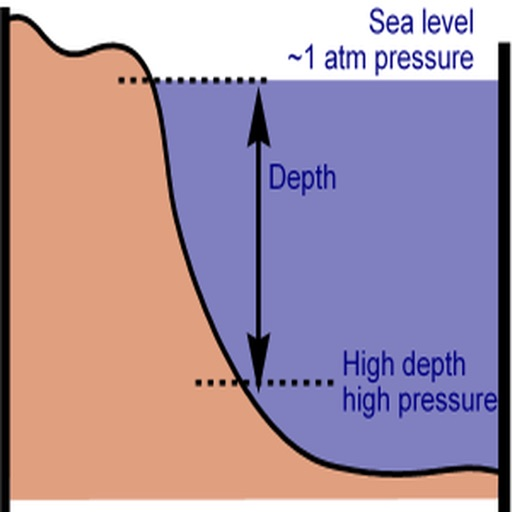## Assuming the density of sea water to be 1025 kg/m³ (in fact it is slightly variable), pressure increases by 1 atm with each 10 m of depth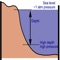# Calculate Pressure In Water

by junjie ren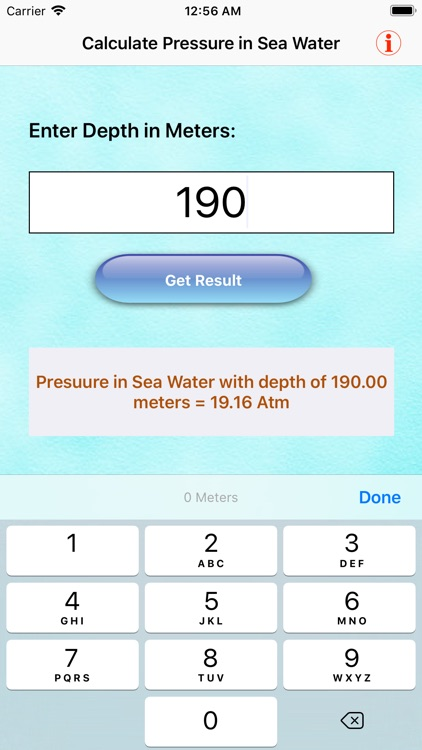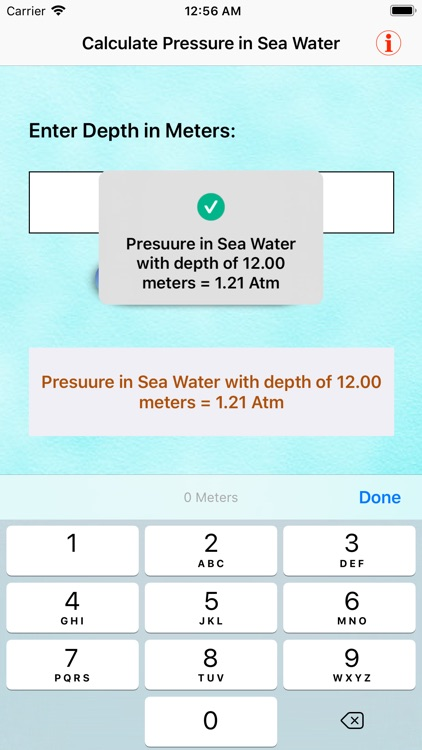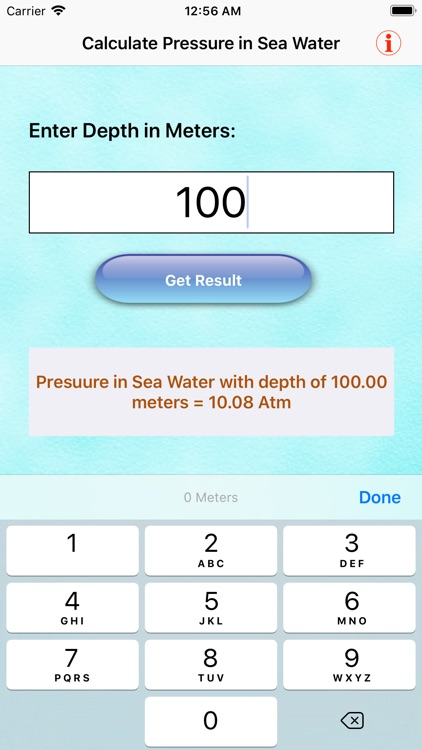Assuming the density of sea water to be 1025 kg/m³ (in fact it is slightly variable), pressure increases by 1 atm with each 10 m of depth. Diver must alter their habits according to the pressure they experience.This app gives the total pressure (not just the pressure due to the water), so it includes the 1 atm ambient air pressure at sea level.This app helps us to study depth of water relationship with Pressure.It is difficult to understand concept of pressure for sea water for a layman.As soon as we move more deeper in water sea pressure will increase more.So this app is a Pressure measuring device for Sea Water.Same concept for all fluids.So for students this app helps to open new doors of concept.You will be able to calculate Pressure with depth.### App Details

Version
1.0
Rating
NA
Size
16Mb
Genre
Education Reference
Last updated
January 4, 2020
Release date
January 4, 2020

### App Screenshots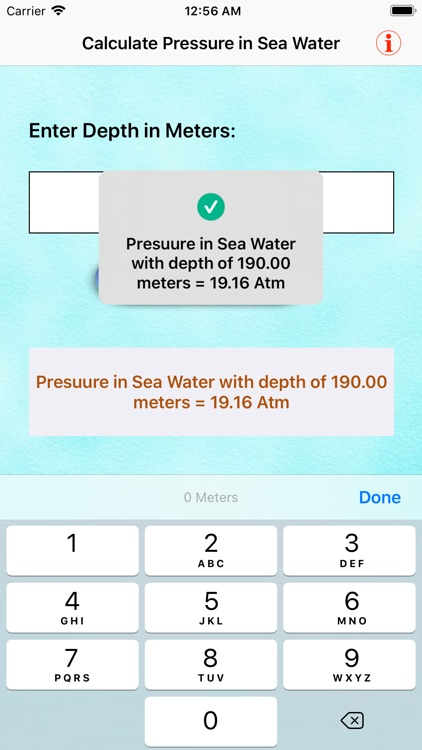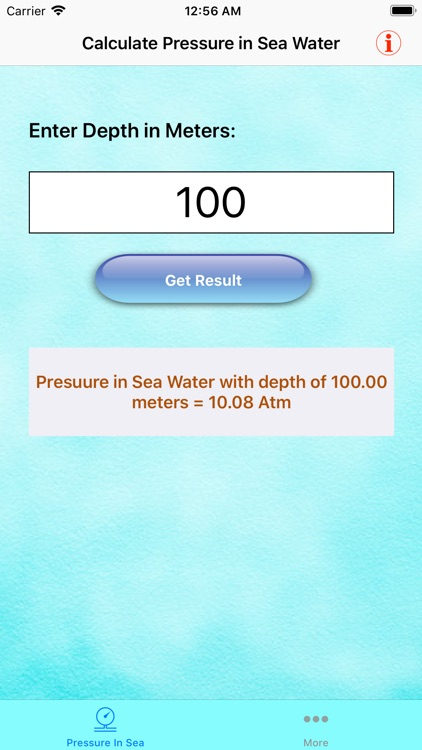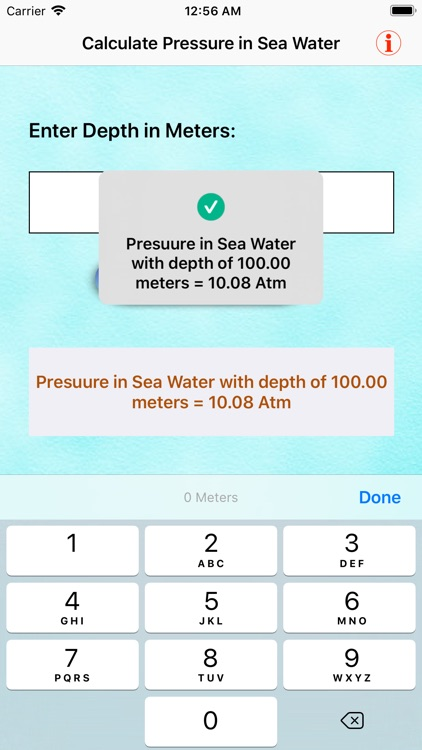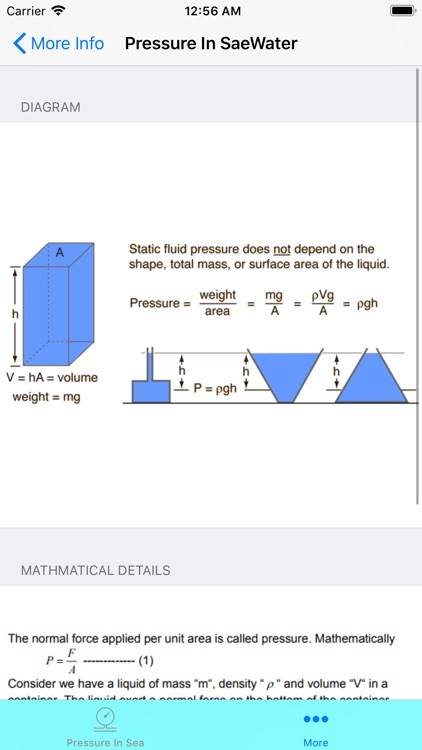### App Store Description

Assuming the density of sea water to be 1025 kg/m³ (in fact it is slightly variable), pressure increases by 1 atm with each 10 m of depth. Diver must alter their habits according to the pressure they experience.This app gives the total pressure (not just the pressure due to the water), so it includes the 1 atm ambient air pressure at sea level.This app helps us to study depth of water relationship with Pressure.It is difficult to understand concept of pressure for sea water for a layman.As soon as we move more deeper in water sea pressure will increase more.So this app is a Pressure measuring device for Sea Water.Same concept for all fluids.So for students this app helps to open new doors of concept.You will be able to calculate Pressure with depth.

This app can solve hydrostatic pressure problems by finding unknown value in hydrostatic equation.
The equation is as follows:
fluid pressure = p × g × h
It states that the pressure difference between two elevations in fluid is the product of elevation change, gravity, and density.
Calculator can solve this equation

- for pressure using known density, height and gravity
- for density using known pressure, height and gravity
- for height using known pressure, density and gravity
- for gravity using known pressure, height and density
All formulas are trivial. The input's default values are as follows:

-density is density of water
-gravity is gravity on Earth

To calculate fluid pressure, use the formula p × g × h = fluid pressure, where p is the density of the liquid, g is the acceleration of gravity, and h is the height of the fluid. Multiply the variables and take the product of the three to solve the equation.

Disclaimer:
AppAdvice does not own this application and only provides images and links contained in the iTunes Search API, to help our users find the best apps to download. If you are the developer of this app and would like your information removed, please send a request to takedown@appadvice.com and your information will be removed.